Next: Summary Up: Applications to Lennard-Jones Clusters Previous: Isomerisation of LJ   Contents

## Internal Diffusion in LJ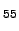We have applied the graph transformation method to study the centre-to-surface atom migration in 55-atom Lennard-Jones cluster. The global potential energy minimum for LJis a Mackay icosahedron, which exhibits special stability and magic number' properties [280,279]. Centre-to-surface and surface-to-centre rates of migration of a tagged atom for this system were considered in previous work . In Reference Wales04 the standard DPS procedure was applied to create and converge an ensemble of paths linking the structure of the global minimum with the tagged atom occupying the central position and structures where tagged atom is placed in sites that lie on fivefold and twofold symmetry axes (Figure 4.15). We have reused this sample in the present work.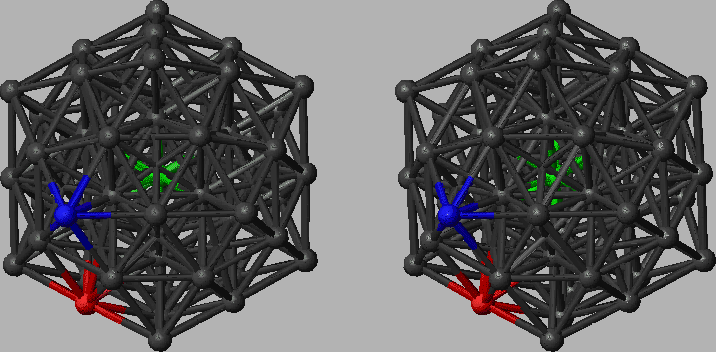The sample contained 9907 minima and 19384 transition states. We excluded transition states that facilitate degenerate rearrangements from consideration. For minima interconnected by more than one transition state we added the rate constants in each direction to calculate the branching probabilities. Four digraph representations were created with minimum degrees of 1, 2, 3 and 4 via iterative removal of the nodes with degrees that did not satisfy the requirement. These digraphs will be referred to as digraphs 1, 2, 3 and 4, respectively. The corresponding parameters are summarised in Table 4.1. Since the cost of the GT method does not depend on temperature we also quote CPU timings for the DGT, SGT and SDGT methods for each of these graphs in the last three columns of Table 4.1. Each digraph contained two source nodes labelled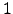and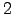and a single sink. The sink node corresponds to the global minimum with the tagged atom in the centre (Figure 4.15). It is noteworthy that the densities of the graphs corresponding to both our samples (LJ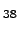and LJ) are significantly lower than the values predicted for a complete sample , which makes the use of sparse-optimised methods even more advantageous. From Table 4.1 it is clear that the SDGT approach is the fastest, as expected; we will use SDGT for all the rate calculations in the rest of this section.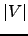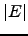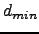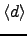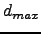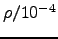.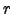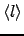.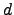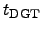.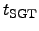.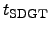. 9843 34871 1 3 . 9 983 3 . 6 10 5 . 71 20 2346 . 1 39 . 6 1 . 36 6603 28392 2 4 . 8 983 6 . 5 9 4 . 86 17 1016 . 1 38 . 9 1 . 33 2192 14172 3 7 . 9 873 29 . 5 4 3 . 63 8 46 . 9 5 . 9 0 . 49 865 7552 4 1 . 9 680 101 . 0 4 3 . 07 7 3 . 1 0 . 8 0 . 12 . . . . . .

For this sample KMC calculations are unfeasible at temperatures lower than about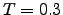(Here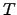is expressed in the units of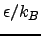). Already for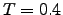the average KMC trajectory length is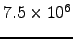(value obtained by averaging over 100 trajectories). In previous work it was therefore necessary to use the DPS formalism, which invokes a steady-state approximation for the intervening minima, to calculate the rate constant at temperatures below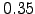. Here we report results that are in direct correspondence with the KMC formulation of the problem, for temperatures as low as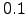.

Figure 4.16 presents Arrhenius plots that we calculated using the SDGT method for this system. The points in the green dataset are the results from seven SDGT calculations at temperatures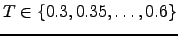conducted for each of the digraphs. The total escape probabilities,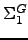and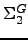, calculated for each of the two sources at the end of the calculation deviated from unity by no more than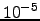. For higher temperatures and smaller digraphs the deviation was smaller, being on the order of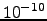for digraph 4 at, and improving at higher temperatures and/or smaller graph sizes.

At temperatures lower than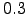the probability deviated by more thandue to numerical imprecision. This problem was partially caused by the round-off errors in evaluation of terms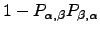, which increase when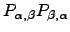approaches unity. These errors can propagate and amplify as the evaluation proceeds. By writing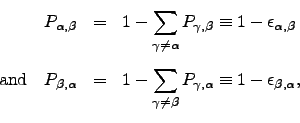(6.44)

and then using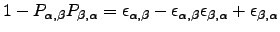(6.45)

we were able to decrease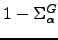by several orders of magnitude at the expense of doubling the computational cost. The SDGT method with probability denominators evaluated in this fashion will be referred to as SDGT1.

Terms of the formapproach zero when nodes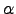and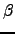become effectively' (i.e. within available precision) disconnected from the rest of the graph. If this condition is encountered in the intermediate stages of the calculation it could also mean that a larger subgraph of the original graph is effectively disconnected. The waiting time for escape if started from a node that belongs to this subgraph tends to infinity. If the probability of getting to such a node from any of the source nodes is close to zero the final answer may still fit into available precision, even though some of the intermediate values cannot. Obtaining the final answer in such cases can be problematic as division-by-zero exceptions may occur.

Another way to alleviate numerical problems at low temperatures is to stop round-off errors from propagation at early stages by renormalising the branching probabilities of affected nodes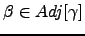after node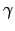is detached. The corresponding check that the updated probabilities of nodeadd up to unity could be inserted after line 33 of Algorithm B.4 (see Appendix B), and similarly for Algorithm B.3. A version of SDGT method with this modification will be referred to as SDGT2.

Both SDGT1 and SDGT2 have similarly scaling overheads relative to the SDGT method. We did not find any evidence for superiority of one scheme over another. For example, the SDGT calculation performed for digraph 4 at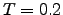yielded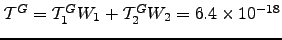, and precision was lost as bothandwere less than. The SDGT1 calculation resulted in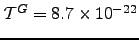and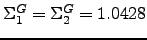, while the SDGT2 calculation produced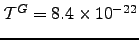with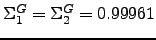. The CPU time required to transform this graph using our implementations of the SDGT1 and SDGT2 methods was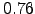and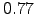seconds, respectively.

To calculate the rates at temperatures in the interval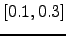reliably we used an implementation of the SDGT2 method compiled with quadruple precision (SDGT2Q) (note that the architecture is the same as in other benchmarks, i.e. with 32 bit wide registers). The points in the blue dataset in Figure 4.16 are the results from 4 SDGT2Q calculations at temperatures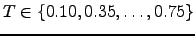.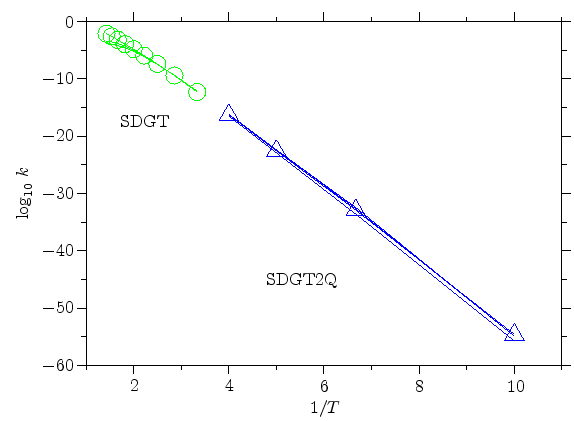By using SDGT2Q we were also able to improve on the low-temperature results for LJpresented in the previous section. The corresponding data is shown in blue in Figure 4.14.Next: Summary Up: Applications to Lennard-Jones Clusters Previous: Isomerisation of LJ   Contents
Semen A Trygubenko 2006-04-10